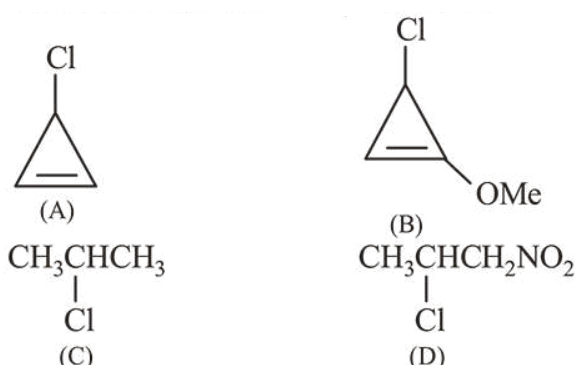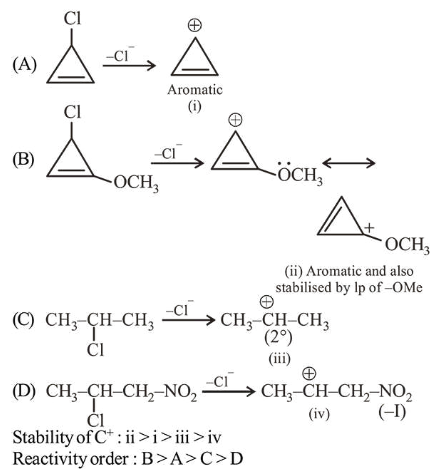# The decreasing order of reactivity of the followingQuestion:

The decreasing order of reactivity of the following organic molecules towards $\mathrm{AgNO}_{3}$ solution is :1. $(\mathrm{C})>(\mathrm{D})>(\mathrm{A})>(\mathrm{B})$

2. $(\mathrm{A})>(\mathrm{B})>(\mathrm{D})>(\mathrm{C})$

3. $(\mathrm{A})>(\mathrm{B})>(\mathrm{C})>(\mathrm{D})$

4. $(\mathrm{B})>(\mathrm{A})>(\mathrm{C})>(\mathrm{D})$

Correct Option: , 4

Solution:

Given reaction is $S_{N}$ 1 reaction. In $S_{N}$ l reaction Rate of reaction $\propto$ Stability of $\mathrm{C}^{+}$